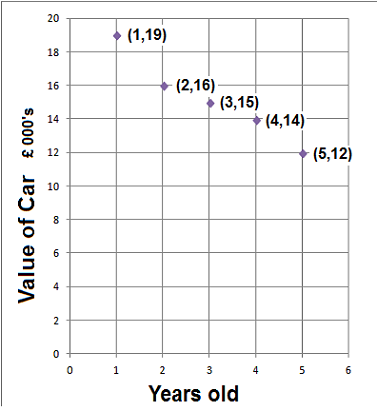### Scatter Diagrams

Scatter diagrams show the link between two variables. The variables are plotted as coordinates. This link shows the correlation (relationship) between the two variables.

There are 3 types of correlation

 1. Positive correlation As the value of the first variable increases so does the second 2. Negative correlation As the value of the first variable increases the second decreases 3. No correlation No linear relationship between the variables

#### Example 1.   Plot a scatter diagram of this table, what type of correlation is there?

 Height m 1 2 3 4 5 6 Weight kg 4 5 6 7 9 9There is a Postive Correlation

#### Example 2.   Plot a scatter diagram of this table, what type of correlation is there?

 Age in Years 1 2 3 4 5 Value of Car£000s 19 16 15 14 12There is a Negative Correlation

#### Example 3.   Plot a scatter diagram of the two tests, what type of correlation is there?

 Art 6 9 1 2 6 2 10 Maths 5 19 10 7 12 18 12There is No Correlationto: